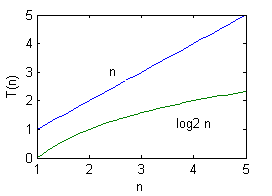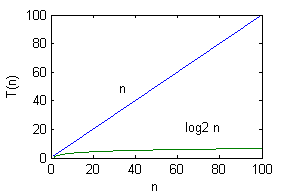### Recitation 06

#### Binary Search

• Binary search has a rather illustrious past. First published in 1946, there actually wasn't a bug-free implementation until 1962. Furthermore, most common implementations today still contain a bug!
• Implementation
• Note that linear search (sorted) and binary serach have the exact same preconditions and postconditions! This means that, suppose we had a program the uses this linear search function extensively, we could replace all of the calls with binary search without worrying about any changes in the program's behavior (as long as we're not assuming anything more about their behavior than what's in the annotations).
• Note that the input array must be sorted. We'll quickly see that the function wouldn't work otherwise.
• Here's an example of searching through the list
$$0\quad 1\quad 2\quad 3\quad 4\quad 5\quad 6\quad 7\quad 8\quad 9\quad$$ Initially, lower = 0, upper = 10, and mid = 0 + (10 - 0) / 2 = 5. So,
 0 1 2 3 4 5 6 7 8 9 $\uparrow$ $\uparrow$ $\uparrow$ l m u
Suppose we are searching for 6. Then the steps through the iterations would look like this:  0 1 2 3 4 5 6 7 8 9 a[mid] < x $\uparrow$ $\uparrow$ $\uparrow$ 0 1 2 3 4 5 6 7 8 9 a[mid] > x $\uparrow$ $\uparrow$ $\uparrow$ 0 1 2 3 4 5 6 7 8 9 a[mid] > x $\uparrow$ $\uparrow$ $\uparrow$ 0 1 2 3 4 5 6 7 8 9 a[mid] == x $\uparrow \uparrow$ $\uparrow$
• Exercise: Consider the case where the desired element is not present and walk through the steps, like above.
• A rough proof for this function was done in class. Here are some of the important things to keep in mind for this proof:
• Remember that the loop invariants must always hold. Notice that the second and third invariant have an "exception" built in to prevent an out of bounds array access, using short-circuit evaluation. Why is it okay to check the right half of the invariant in these cases?
• The loop invariants and the negated loop condition must imply the postcondition. We can always plug the values from when we leave the loop into the loop invariant to bridge the gap to the postcondition.
• Why do we know that the loop must terminate? Well, if we find the element we're looking for, we return immediately. If the element does not exist, in each iteration, either lower gets larger or upper gets smaller. In other words, they're getting closer together and there is no case where neither is changed.
• If there are multiple occurances of the element we're looking for in the array, which one will we get? Actually, there's no guarantee which one the result will be.

#### Counting Operations

For this example, we will consider the code contained in swap.c0

We first notice that the loop runs $n - 1$ times. In each iteration, we have two operations--one checking the loop condition and another incrementing $i$--in addition to the function call. Furthermore, there is one extra op that occurs once, initially setting $i = 0$. Lastly, swap() has 3 ops. Therefore, we can refer to the number of operations on an input of size $n$ as $T(n) = (n - 1)(3 + 2) + 1 = 5n - 4$.

Now, that was pretty tedious, right? Especially for any larger function, counting operations would be a nightmare. Well, does the number of ops really matter? The more important thing to take away from this is that the number of ops has some dependence on $n$. In fact, we could say that $T(n) \propto n$ ($\propto$: is proportional to). Going back to our equation before, how does the number of ops change as I change $n$? If $n = 10$ then $T(n) = 5(10) - 4 = 46$. If we increase to $n = 20$ then $T(n) = 5(20) - 4 = 96$. So, the number of operations roughly doubled, which makes sense.

Now, let's consider binary search again. In iteration of the loop, we effectively cut the number of elements between upper and lower in half each time. So, for binary search, it doesn't seem like $T(n) \propto n$, since most of the elements are never visited. It turns out that by cutting half of the remaining elements out each time, $T(n) \propto log_2 n$. Consider how this affects the total number of operations as $n$ varies for a small interval (left) and a large interval (right).On the small interval, there is a noticeable difference, but it's not too impressive. However, on the larger interval the difference is quite dramatic. So, what can we conclude about how using linear search compares to binary search? We will continue exploring ways to analyze runtime next week.

#### isqrt()

We also reasoned over the invariants in isqrt(). Here is the file, if you would like to try the proof again on your own.

If you have any comments or see any errors, please let me know.